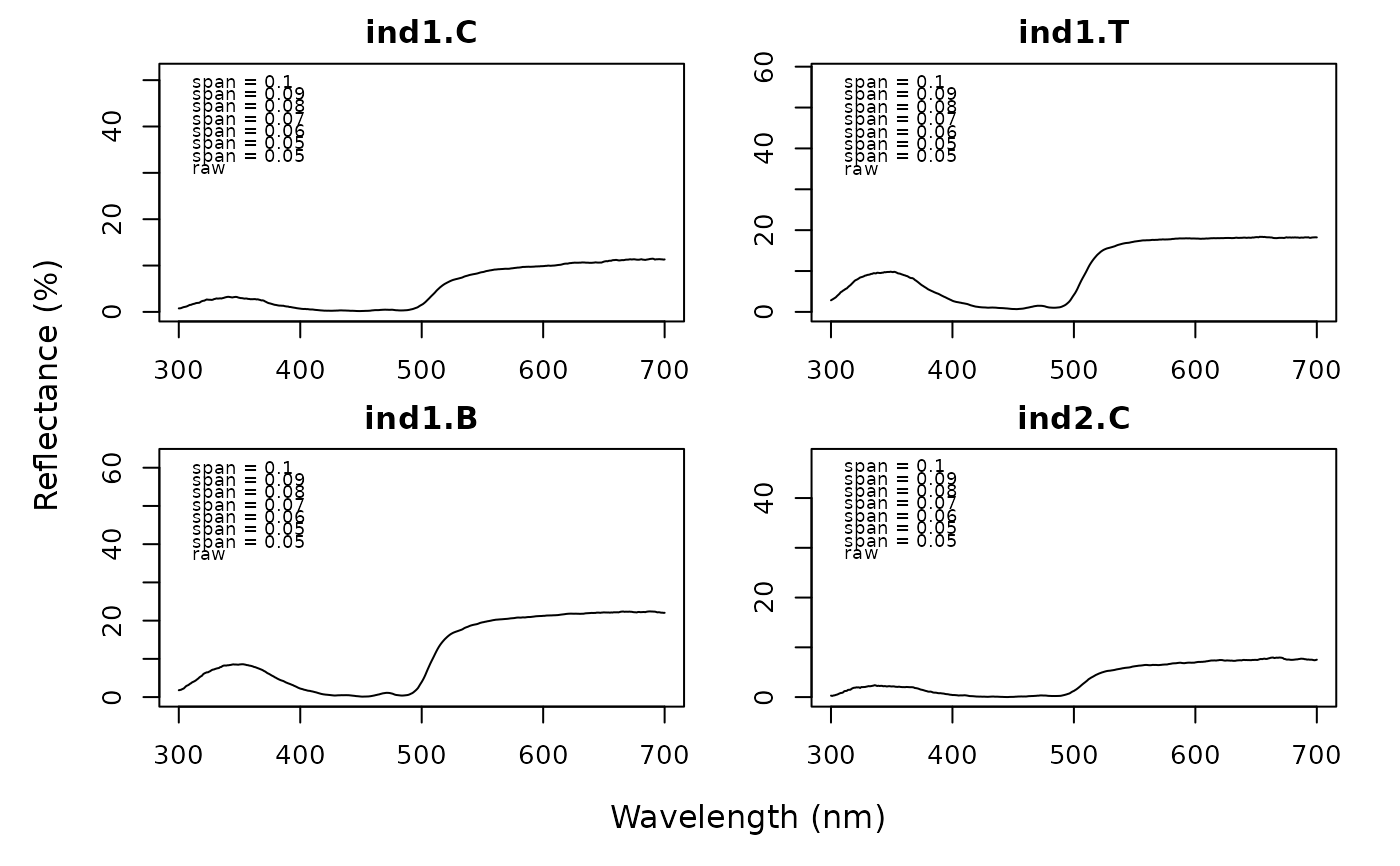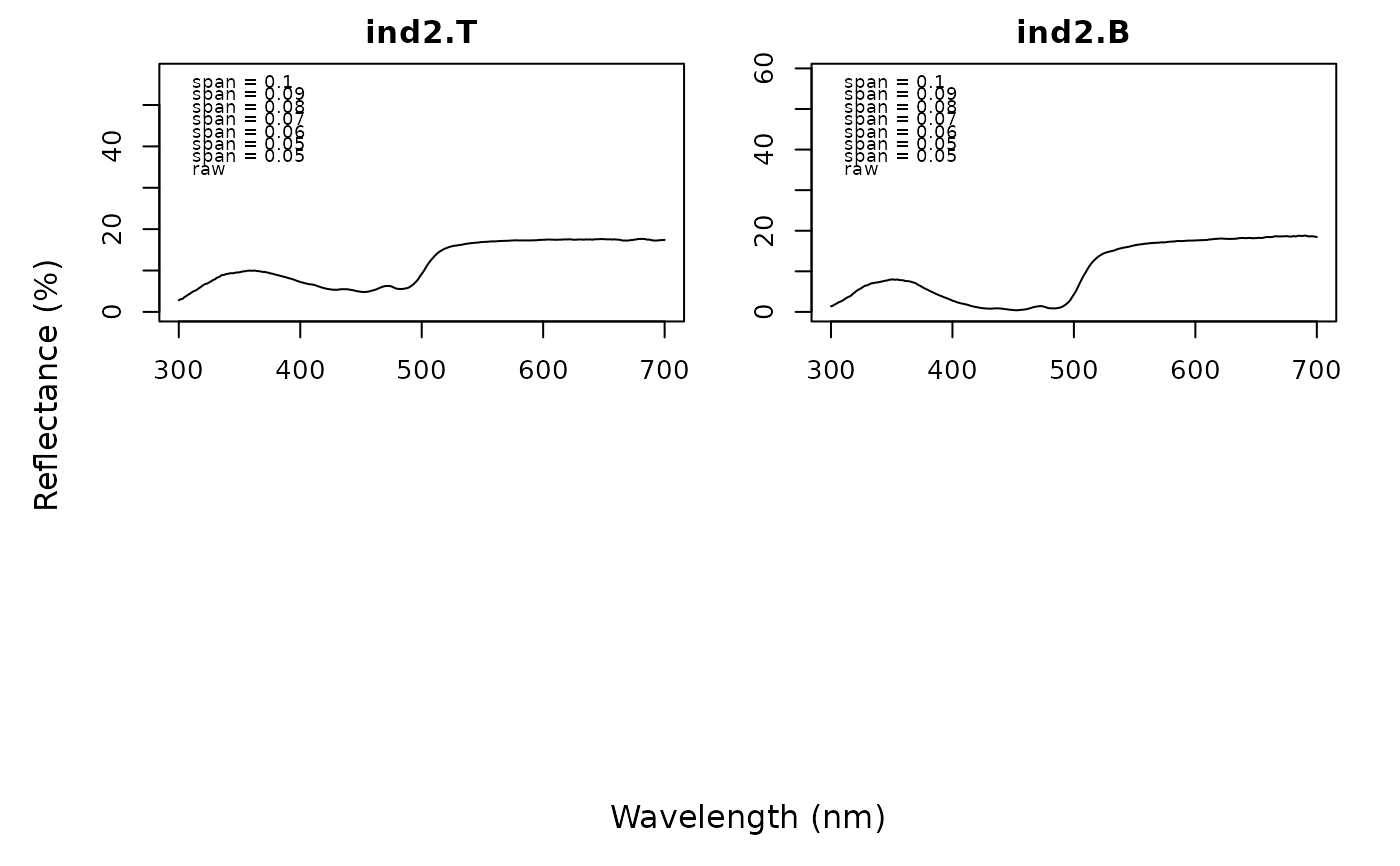Plots spectral curves with various levels of loess smoothing to help decide which loess parameters are best for subsequently smoothing the data (e.g. via procspec()).

plotsmooth(
rspecdata,
minsmooth = 0.05,
maxsmooth = 0.2,
curves = 5,
specnum = "ALL",
)

## Arguments

rspecdata

(required) a data frame, possibly of class rspec, which contains a column containing a wavelength range, named 'wl', and spectra data in remaining columns.

minsmooth

the minimum f value of the loess function to visualize (defaults to 0.05).

maxsmooth

the maximum f value of the loess function to visualize (defaults to 0.20).

curves

the number of curves to display on the same plot (defaults to 5).

specnum

the number of spectral curves, from the data frame, to visualize (defaults to ALL).

logical. if TRUE, asks for user input before changing plot pages

## Value

Series of plot with curves processed with varying level of loess smoothing

procspec()

## Author

Pierre-Paul Bitton bittonp@uwindsor.ca

## Examples

# Load reflectance spectra
data(sicalis)

# Visualise the spectral reflectance curves across a range of smoothing levels
plotsmooth(sicalis, minsmooth = 0.05, maxsmooth = 0.1, curves = 7, specnum = 6)